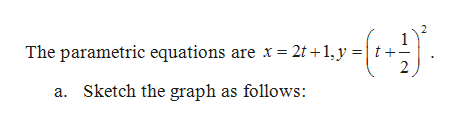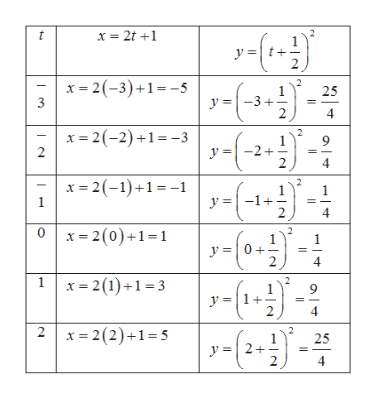# The question says:"A pair of parametric equations is given. a.) Sketch the curve represented by the parametric equations. b.) Find a rectangular-coordinate equation for the curve by eliminating the parameter. x=2t+1, y=(t+ 1/2)2

Question

The question says:

"A pair of parametric equations is given. a.) Sketch the curve represented by the parametric equations. b.) Find a rectangular-coordinate equation for the curve by eliminating the parameter.

x=2t+1, y=(t+ 1/2)2

check_circle

Step 1

Given:help_outlineImage TranscriptioncloseThe parametric equations are x 2t +1,y 2 Sketch the graph as follows: a. fullscreen
Step 2

The table is obtained as follows:help_outlineImage Transcriptionclosex 2-3)+1-5 25 y=-3 + 2 3 4 x 2(-2)1-3 2 9 2 4 x 2)+ y-1 1 2. 4 0x2(0)+1-1 4 12(1)3 9 4 2 2(2)+15 y2-2 25 4 fullscreen
Step 3

The sketch of the parame...

### Want to see the full answer?

See Solution

#### Want to see this answer and more?

Solutions are written by subject experts who are available 24/7. Questions are typically answered within 1 hour.*

See Solution
*Response times may vary by subject and question.
Tagged in

### Functions